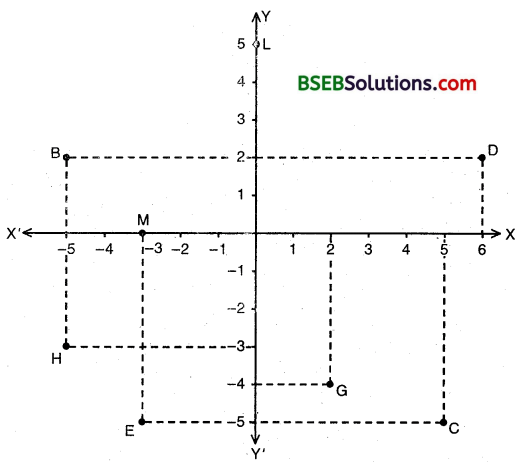# HSSlive: Plus One & Plus Two Notes & Solutions for Kerala State Board

## BSEB Class 9 Maths Chapter 3 Coordinate Geometry Ex 3.2 Textbook Solutions PDF: Download Bihar Board STD 9th Maths Chapter 3 Coordinate Geometry Ex 3.2 Book AnswersBSEB Class 9 Maths Chapter 3 Coordinate Geometry Ex 3.2 Textbook Solutions PDF: Download Bihar Board STD 9th Maths Chapter 3 Coordinate Geometry Ex 3.2 Book Answers

BSEB Class 9th Maths Chapter 3 Coordinate Geometry Ex 3.2 Textbooks Solutions and answers for students are now available in pdf format. Bihar Board Class 9th Maths Chapter 3 Coordinate Geometry Ex 3.2 Book answers and solutions are one of the most important study materials for any student. The Bihar Board Class 9th Maths Chapter 3 Coordinate Geometry Ex 3.2 books are published by the Bihar Board Publishers. These Bihar Board Class 9th Maths Chapter 3 Coordinate Geometry Ex 3.2 textbooks are prepared by a group of expert faculty members. Students can download these BSEB STD 9th Maths Chapter 3 Coordinate Geometry Ex 3.2 book solutions pdf online from this page.

## Bihar Board Class 9th Maths Chapter 3 Coordinate Geometry Ex 3.2 Books Solutions

 Board BSEB Materials Textbook Solutions/Guide Format DOC/PDF Class 9th Subject Maths Chapter 3 Coordinate Geometry Ex 3.2 Chapters All Provider Hsslive

## How to download Bihar Board Class 9th Maths Chapter 3 Coordinate Geometry Ex 3.2 Textbook Solutions Answers PDF Online?

2. Click on the Bihar Board Class 9th Maths Chapter 3 Coordinate Geometry Ex 3.2 Answers.
3. Look for your Bihar Board STD 9th Maths Chapter 3 Coordinate Geometry Ex 3.2 Textbooks PDF.
4. Now download or read the Bihar Board Class 9th Maths Chapter 3 Coordinate Geometry Ex 3.2 Textbook Solutions for PDF Free.

## BSEB Class 9th Maths Chapter 3 Coordinate Geometry Ex 3.2 Textbooks Solutions with Answer PDF Download

Find below the list of all BSEB Class 9th Maths Chapter 3 Coordinate Geometry Ex 3.2 Textbook Solutions for PDF’s for you to download and prepare for the upcoming exams:

## BSEB Bihar Board Class 9th Maths Solutions Chapter 3 Coordinate Geometry Ex 3.2

Question 1.
Write the answer of each of the following questions :

1. What is the name of horizontal and the vertical lines drawn to determine the position of any point in the Cartesian plane?
2. What is the name of each part of the plane formed by these two lines?
3. Write the name of the point where these two lines intersect.

Solution:

1. The name of horizontal and vertical lines drawn to divide the plane into four parts is rectangular axes/coordinate axes.
2. The name of each part of the plane divided by axes is quadrant.
3. The axes intersects at the origin.

Question 2.
See figure, and write the following :

1. The coordinates of B.
2. The coordinates of C.
3. The point identified by the coordinates (- 3, – 5).
4. The point identified by the coordinates (2, – 4).
5. The abscissa of the point D.
6. The ordinate of the point H.
7. The coordinates of point L.
8. The coordinates of the point M.Solution:
Clearly, from the figure :

1. The coordinates of B are (- 5, 2).
2. The coordinates of C are (5, – 5).
3. The coordinates (- 3, – 5) are identified by the point E
4. The coordinates (2, – 4) are identified by the point G
5. The abscissa of the point D is 6.
6. The ordinate of the point H is – 3.
7. The coordinates of the point L are (0, 5)
8. The coordinates of the point M are (- 3, 0).

## Bihar Board Class 9th Maths Chapter 3 Coordinate Geometry Ex 3.2 Textbooks for Exam Preparations

Bihar Board Class 9th Maths Chapter 3 Coordinate Geometry Ex 3.2 Textbook Solutions can be of great help in your Bihar Board Class 9th Maths Chapter 3 Coordinate Geometry Ex 3.2 exam preparation. The BSEB STD 9th Maths Chapter 3 Coordinate Geometry Ex 3.2 Textbooks study material, used with the English medium textbooks, can help you complete the entire Class 9th Maths Chapter 3 Coordinate Geometry Ex 3.2 Books State Board syllabus with maximum efficiency.

## FAQs Regarding Bihar Board Class 9th Maths Chapter 3 Coordinate Geometry Ex 3.2 Textbook Solutions

#### Can we get a Bihar Board Book PDF for all Classes?

Yes you can get Bihar Board Text Book PDF for all classes using the links provided in the above article.

## Important Terms

Bihar Board Class 9th Maths Chapter 3 Coordinate Geometry Ex 3.2, BSEB Class 9th Maths Chapter 3 Coordinate Geometry Ex 3.2 Textbooks, Bihar Board Class 9th Maths Chapter 3 Coordinate Geometry Ex 3.2, Bihar Board Class 9th Maths Chapter 3 Coordinate Geometry Ex 3.2 Textbook solutions, BSEB Class 9th Maths Chapter 3 Coordinate Geometry Ex 3.2 Textbooks Solutions, Bihar Board STD 9th Maths Chapter 3 Coordinate Geometry Ex 3.2, BSEB STD 9th Maths Chapter 3 Coordinate Geometry Ex 3.2 Textbooks, Bihar Board STD 9th Maths Chapter 3 Coordinate Geometry Ex 3.2, Bihar Board STD 9th Maths Chapter 3 Coordinate Geometry Ex 3.2 Textbook solutions, BSEB STD 9th Maths Chapter 3 Coordinate Geometry Ex 3.2 Textbooks Solutions,
Share: